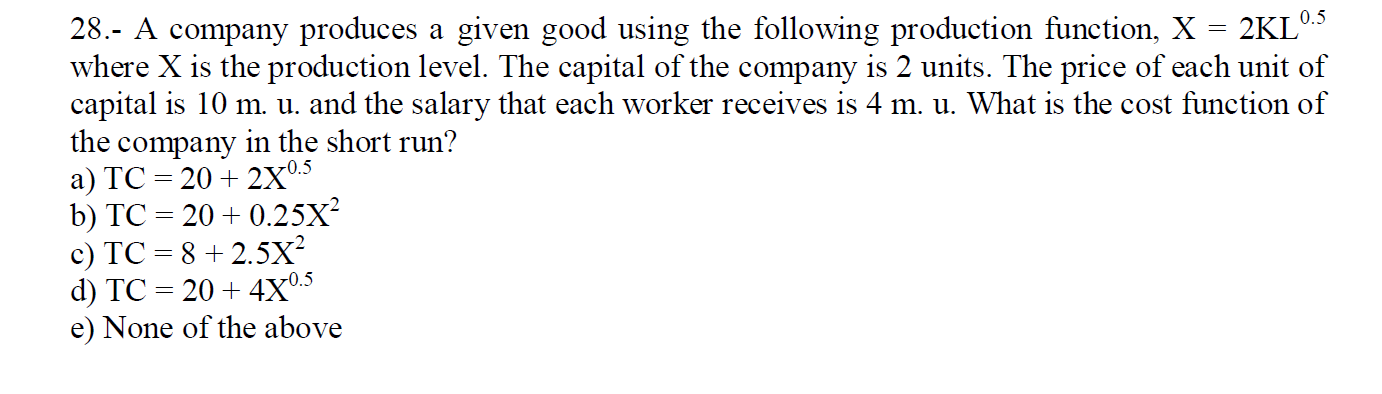# (Solved): Please give step by step solution 28.- A company produces a given good using the following producti ...Please give step by step solution

28.- A company produces a given good using the following production function, $$\mathrm{X}=2 \mathrm{KL}{ }^{0.5}$$ where $$\mathrm{X}$$ is the production level. The capital of the company is 2 units. The price of each unit of capital is $$10 \mathrm{~m}$$. u. and the salary that each worker receives is $$4 \mathrm{~m}$$. u. What is the cost function of the company in the short run? a) $$\mathrm{TC}=20+2 \mathrm{X}^{0.5}$$ b) $$\mathrm{TC}=20+0.25 \mathrm{X}^{2}$$ c) $$\mathrm{TC}=8+2.5 \mathrm{X}^{2}$$ d) $$\mathrm{TC}=20+4 \mathrm{X}^{0.5}$$ e) None of the above

We have an Answer from Expert